# The SURVEYFREQ Procedure

#### Rao-Scott Likelihood Ratio Chi-Square Test

The Rao-Scott likelihood ratio chi-square test is a design-adjusted version of the likelihood ratio test, which involves ratios of observed and expected frequencies. For details about design-adjusted chi-square tests, see Lohr (2010, Section 10.3.2), Rao and Scott (1981, 1984, 1987); Thomas, Singh, and Roberts (1996).

PROC SURVEYFREQ provides a first-order Rao-Scott likelihood ratio test by default. If you specify the LRCHISQ(SECONDORDER) option, PROC SURVEYFREQ provides a second-order (Satterthwaite) likelihood ratio chi-square test.

The procedure computes the Rao-Scott likelihood ratio test by applying design adjustments to the weighted likelihood ratio statistic that is based on estimated totals. This computation is identical to the Rao-Scott chi-square test computation except that it uses the likelihood ratio statistic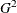in place of the Pearson chi-square statistic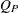. See the section Rao-Scott Chi-Square Test for details.

##### One-Way Tables

For one-way tables, the LRCHISQ option provides a Rao-Scott (design-based) goodness-of-fit test for one-way tables. By default, this is a test for the null hypothesis of equal proportions. If you specify null hypothesis proportions in the TESTP= option, the goodness-of-fit test uses the specified proportions.

The Rao-Scott likelihood ratio test uses the likelihood ratio statistic that is based on the estimated totals,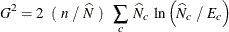where n is the sample size,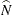is the estimated overall total,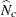is the estimated total for level c, and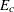is the expected total for level c under the null hypothesis. For the null hypothesis of equal proportions, the expected total for each level equals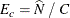For specified null proportions, the expected total for level c equals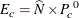where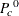is the null proportion that you specify for level c.

The computation of the Rao-Scott likelihood ratio test for one-way tables usesin place ofin the Rao-Scott chi-square test computation and is otherwise identical to the chi-square test computation. See the sections First-Order Test and Second-Order Test for details.

If you specify the LRCHISQ(MODIFIED) option, PROC SURVEYFREQ computes the design corrections by using null hypothesis proportions instead of proportion estimates. By default, null hypothesis proportions are equal proportions for all levels of the one-way table. Alternatively, you can specify null proportion values in the TESTP= option.

##### Two-Way Tables

For two-way tables, the LRCHISQ option provides a Rao-Scott (design-based) test of association between the row and column variables.

The Rao-Scott likelihood ratio test uses the likelihood ratio statistic that is based on the estimated totals,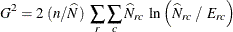where n is the sample size,is the estimated overall total,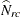is the estimated total for table cell (r, c), and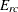is the expected total for cell (r, c) under the null hypothesis of no association. The expected total for cell (r, c) equals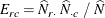The computation of the Rao-Scott likelihood ratio test for two-way tables usesin place ofin the Rao-Scott chi-square test computation and is otherwise identical to the chi-square test computation. See the sections First-Order Test and Second-Order Test for details.

By default for a first-order test, PROC SURVEYFREQ computes the design correction from proportion estimates. If you specify the LRCHISQ(MODIFIED) option for a first-order test, the procedure computes the design correction from null hypothesis proportions.

Second-order tests, which you request by specifying the LRCHISQ(SECONDORDER) option, are computed by applying both first-order and second-order design corrections to the weighted likelihood ratio statistic. For second-order tests for two-way tables, PROC SURVEYFREQ always uses null hypothesis proportions to compute both the first-order and second-order design corrections.